Test: Areas Related to Circles - 2

# Test: Areas Related to Circles - 2

Test Description

## 10 Questions MCQ Test Mathematics (Maths) Class 10 | Test: Areas Related to Circles - 2

Test: Areas Related to Circles - 2 for Class 10 2022 is part of Mathematics (Maths) Class 10 preparation. The Test: Areas Related to Circles - 2 questions and answers have been prepared according to the Class 10 exam syllabus.The Test: Areas Related to Circles - 2 MCQs are made for Class 10 2022 Exam. Find important definitions, questions, notes, meanings, examples, exercises, MCQs and online tests for Test: Areas Related to Circles - 2 below.
Solutions of Test: Areas Related to Circles - 2 questions in English are available as part of our Mathematics (Maths) Class 10 for Class 10 & Test: Areas Related to Circles - 2 solutions in Hindi for Mathematics (Maths) Class 10 course. Download more important topics, notes, lectures and mock test series for Class 10 Exam by signing up for free. Attempt Test: Areas Related to Circles - 2 | 10 questions in 10 minutes | Mock test for Class 10 preparation | Free important questions MCQ to study Mathematics (Maths) Class 10 for Class 10 Exam | Download free PDF with solutions
 1 Crore+ students have signed up on EduRev. Have you?
Test: Areas Related to Circles - 2 - Question 1

### The area of a sector of a circle bounded by an arc of length 5π cm is equal to 20π cm2, then its radius is

Test: Areas Related to Circles - 2 - Question 2

### Area of the largest triangle that can be inscribed in a semi-circle of radius r units is

Detailed Solution for Test: Areas Related to Circles - 2 - Question 2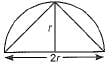Area of triangle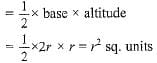Test: Areas Related to Circles - 2 - Question 3

### The ratio of area of two circles whose ratio of circumference is 3:1 will be

Test: Areas Related to Circles - 2 - Question 4

Match the columns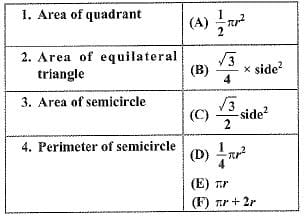Detailed Solution for Test: Areas Related to Circles - 2 - Question 4

Formulae

Test: Areas Related to Circles - 2 - Question 5

It is proposed to build a single circular park equal in area to the sum of areas of two circular parks of diameters 16 m and 12 m in a locality. The radius of the new park would be

Test: Areas Related to Circles - 2 - Question 6

ABC is an equilateral triangle. The area of the shaded region if the radius of each of the circle is 1 cm, is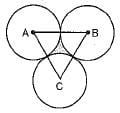Detailed Solution for Test: Areas Related to Circles - 2 - Question 6

Side of triangle = 2 cm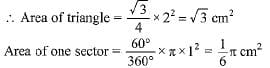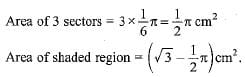Test: Areas Related to Circles - 2 - Question 7

The area of the square that can be inscribed in a circle of radius 8 cm is

Test: Areas Related to Circles - 2 - Question 8

ABCDEF is any hexagon with different vertices A, B, C, D, E and F as the centres of circles with same radius r are drawn. The area of the shaded portion is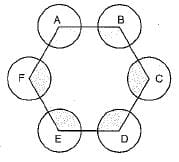Detailed Solution for Test: Areas Related to Circles - 2 - Question 8

Internal angles of a regular Hexagon = 120°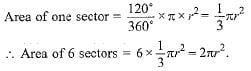Test: Areas Related to Circles - 2 - Question 9

The diameter of a circle whose area is equal to the sum of the areas of the two circles of radii 24 cm and 7 cm is

Test: Areas Related to Circles - 2 - Question 10

The diameter of a wheel is 1 m. The number of revolutions it will make to travel a distance of 22 km will be

## Mathematics (Maths) Class 10

53 videos|369 docs|138 tests
 Use Code STAYHOME200 and get INR 200 additional OFF Use Coupon Code
Information about Test: Areas Related to Circles - 2 Page
In this test you can find the Exam questions for Test: Areas Related to Circles - 2 solved & explained in the simplest way possible. Besides giving Questions and answers for Test: Areas Related to Circles - 2, EduRev gives you an ample number of Online tests for practice

## Mathematics (Maths) Class 10

53 videos|369 docs|138 tests